Question
This is from a Study of Buffer Solutions and pH of Salt Solutions Lab. I calculated Ka to be 3.2*10^-5. Why is my value larger than the standard value?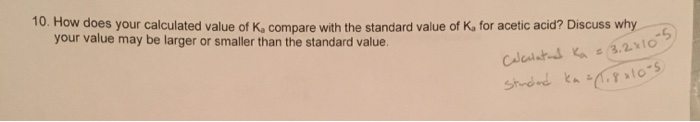Procedure: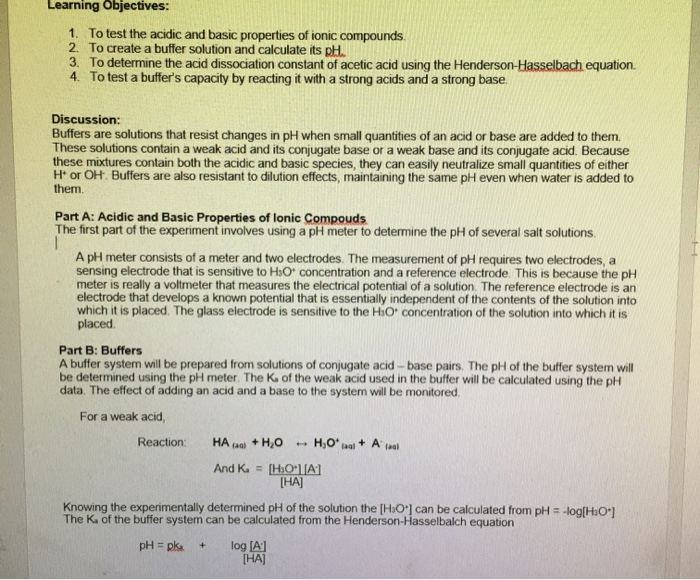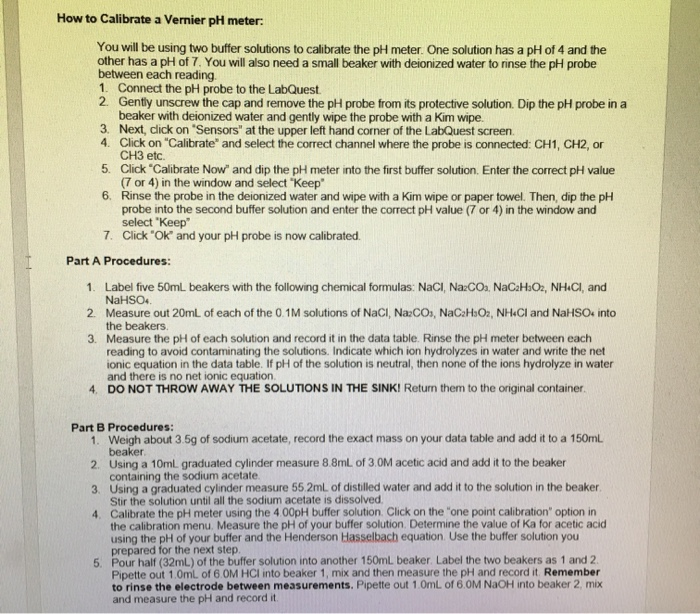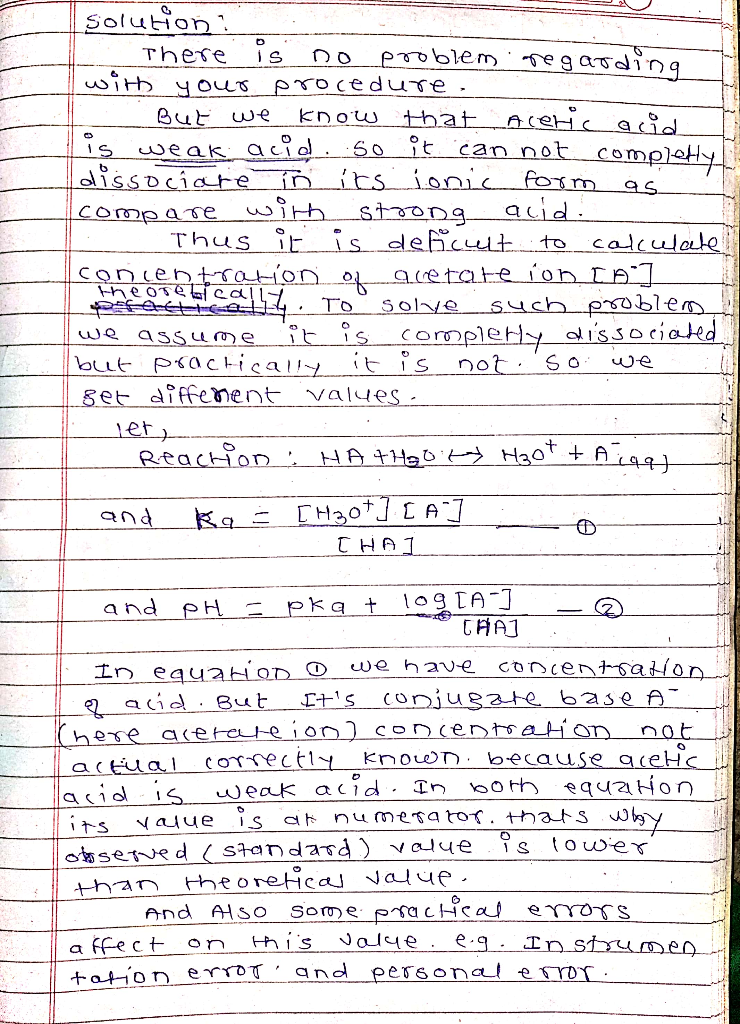#### Earn Coins

Coins can be redeemed for fabulous gifts.

Similar Homework Help Questions
• ### I need help with the problem in the last photo.. I thought I’d post my lab...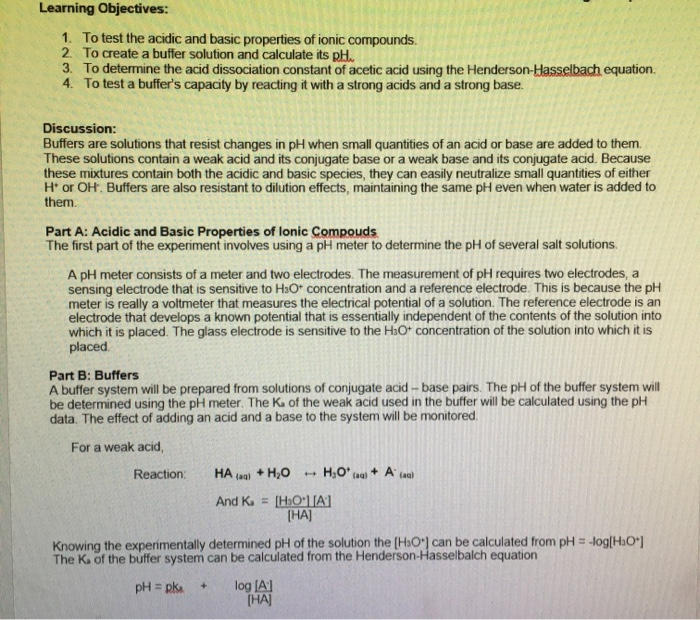I need help with the problem in the last photo.. I thought I’d post my lab explanation and data if that helps you get a better understanding, but it’s just the question at the end. I know I need to use the Henderson Hasselbach equation.. so... 4.70 = pKa + log( [acetate-ion] / [acetic-ion] ) and solve for pKa, then Ka.. but how do I find the concentrations to put in the log fraction? Thanks, in advance! Learning Objectives: 1....

• ### I know some of the measured pH values are a little off due to error in...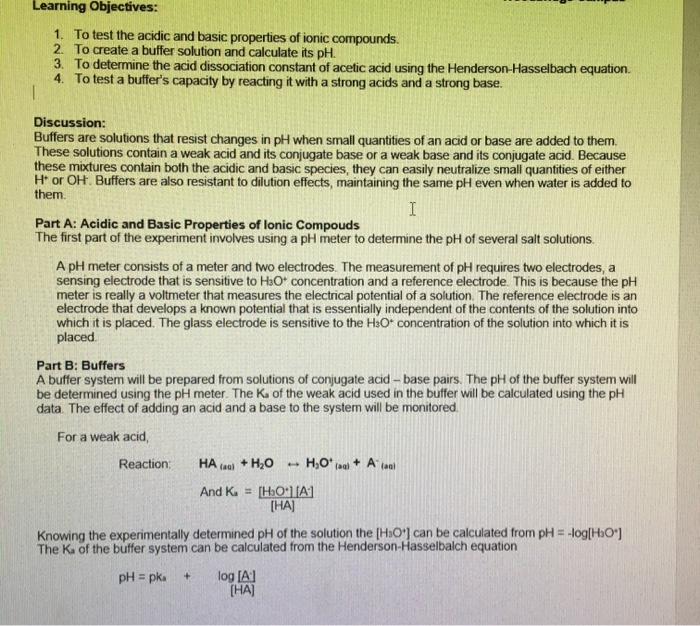I know some of the measured pH values are a little off due to error in the lab, but I need help filling out the “Ion Hydrolyzed” table and then the “Net Ionic Equation” part. I remember doing net ionic equations in General Chemistry I, bit don’t see how it applies here. Thanks, in advance! (i will rate!) Learning Objectives: 1. To test the acidic and basic properties of ionic compounds. 2. To create a buffer solution and calculate its...

• ### Properties of Buffers Lab. I need help with problems 7 & 8. They’re pretty similar, but...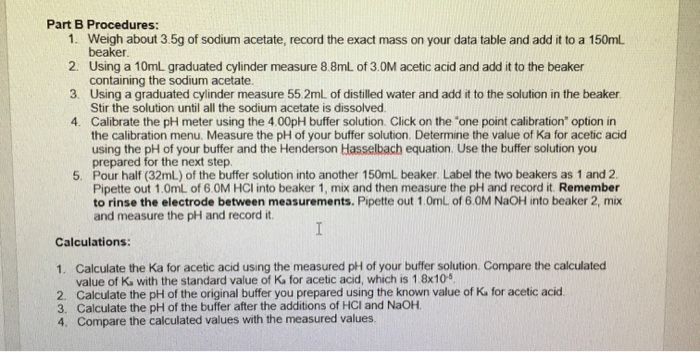Properties of Buffers Lab. I need help with problems 7 & 8. They’re pretty similar, but I’m confused on whether I’m doing them right. I need to calculate the moles of HCl and NaOH and then somehow insert them into the log fraction in the Henderson Hasselbach formula, is that right? Procedure (values are needed in procedure): Measured values during lab that I’ll be comparing #6/7/8 to: Questions I need help with: Part B Procedures: 1. Weigh about 3.5g of...

• ### F. pH of Buffer after the addition of the Strong Base 1. Calculate the expected pH...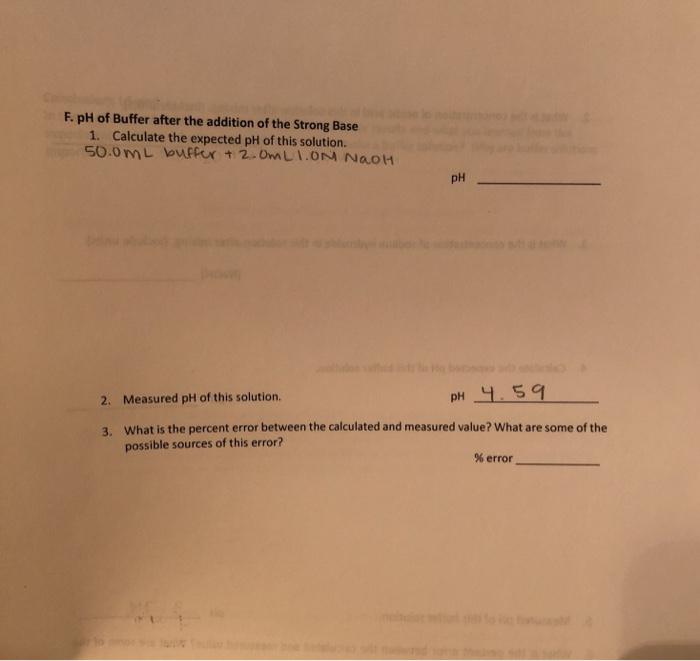F. pH of Buffer after the addition of the Strong Base 1. Calculate the expected pH of this solution. 50.0 mL buffer + 2.0mLION NAOH pH 2. Measured pH of this solution. pH 4.59 3. What is the percent error between the calculated and measured value? What are some of the possible sources of this error? % error pH of Buffer after the addition of the Strong Base: ix. Measure out 50.0 ml the buffer in part D and 2.0...

• ### how would you calculate the expected pH for solution 7, 8, 9, 10, and 11? (I...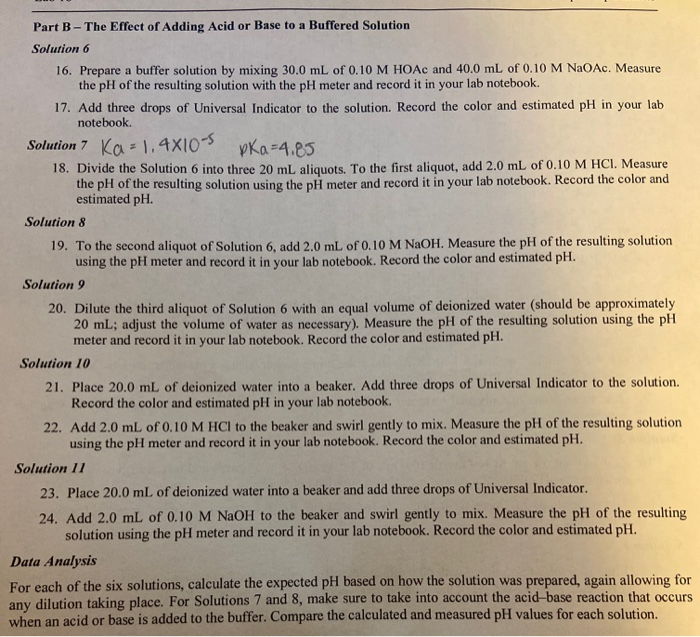how would you calculate the expected pH for solution 7, 8, 9, 10, and 11? (I am confused on how you calculate the dilutions for finding the expected pH too) Ka= 1.4x10^-5 pKa= 4.85 Part B - The Effect of Adding Acid or Base to a Buffered Solution Solution 6 16. Prepare a buffer solution by mixing 30.0 mL of 0.10 M HOAc and 40.0 mL of 0.10 M NaOAc. Measure the pH of the resulting solution with the pH...

• ### I don't understand what calculations I would have made to create the buffer solution from Part...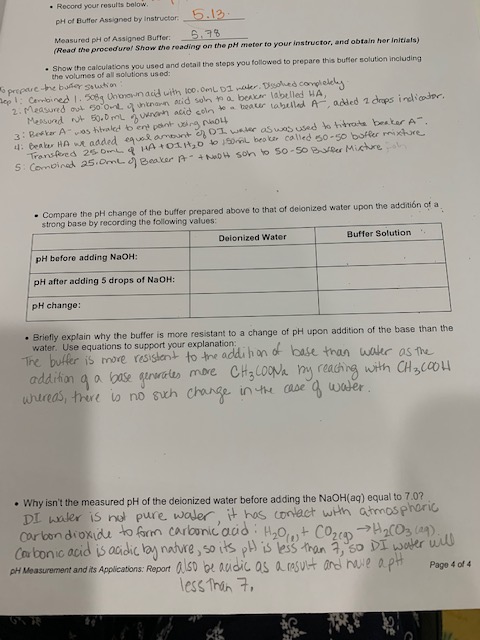I don't understand what calculations I would have made to create the buffer solution from Part D of the lab. I understood the procedures I took to create the solution but when the report asks to show the calculations used to prepare the buffer solution I am not sure other than mixing the volumes indicated in the procedures section. Thank you pH of Butter Assigned by Instructor 5.12. Measured pH of Assigned B r .9 (Read the procedural Show the...

• ### The buffer solution is made of 50ml of 1.0M CH3COOH and 50ml of 1.0M NaCH3COO. Please...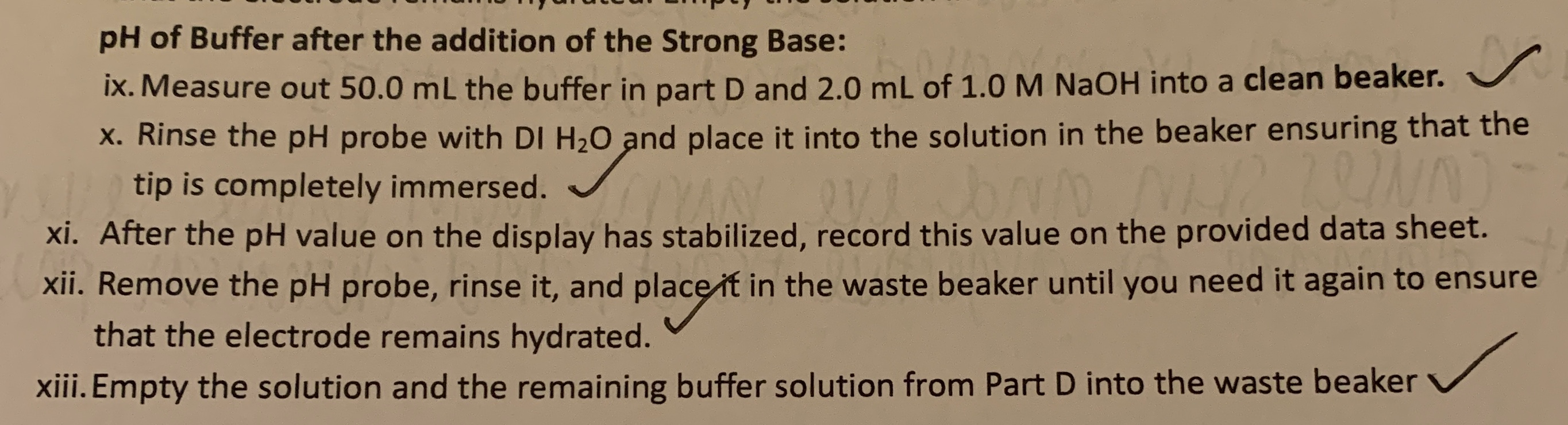The buffer solution is made of 50ml of 1.0M CH3COOH and 50ml of 1.0M NaCH3COO. Please find the expected pH of the buffer after the addition of the strong base, and the percent error between the expected pH and the measured pH of the solution. pH of Buffer after the addition of the Strong Base: ix. Measure out 50.0 mL the buffer in part D and 2.0 mL of 1.0 M NaOH into a clean beaker. X. Rinse the pH...

• ### Base/Acid Ratios in Buffers Just as pH is the negative logarithm of [H3O+], pKa is the...

Base/Acid Ratios in Buffers Just as pH is the negative logarithm of [H3O+], pKa is the negative logarithm of Ka, pKa=?logKa The Henderson-Hasselbalch equation is used to calculate the pH of buffer solutions: pH=pKa+log[base][acid] Notice that the pH of a buffer has a value close to the pKa of the acid, differing only by the logarithm of the concentration ratio [base]/[acid]. Part A Acetic acid has a Ka of 1.8

• ### I need to know if I calculated the acetate buffer correctly & how to calculate the...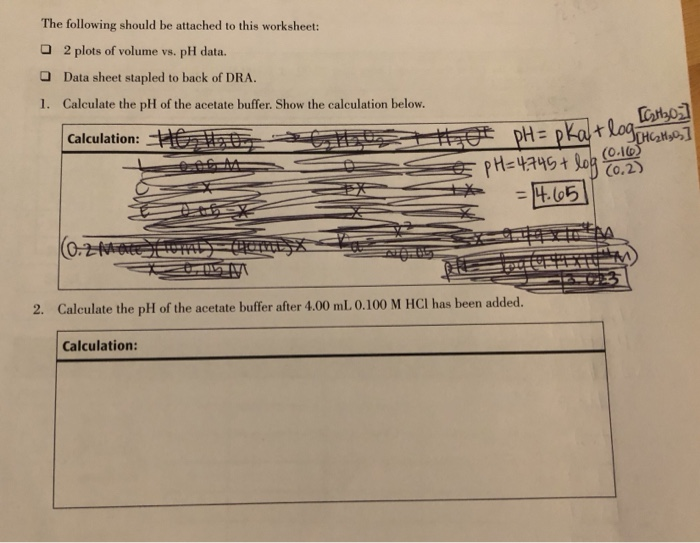I need to know if I calculated the acetate buffer correctly & how to calculate the change in pH when HCl is added... The following should be attached to this worksheet: 2 plots of volume vs. pH data. Data sheet stapled to back of DRA. 1. Calculate the pH of the acetate buffer. Show the calculation below. Calculation: Ho t t 419 [Cathoz ot pH= pkatlog HGH sos] Œ pH=4.745 + log NAH ** = 4.65 (0.2 m m 2....

• ### Please help, I'm so confused!!!! This is due wednesday night!!! i'm gonna fail :(((( pH of...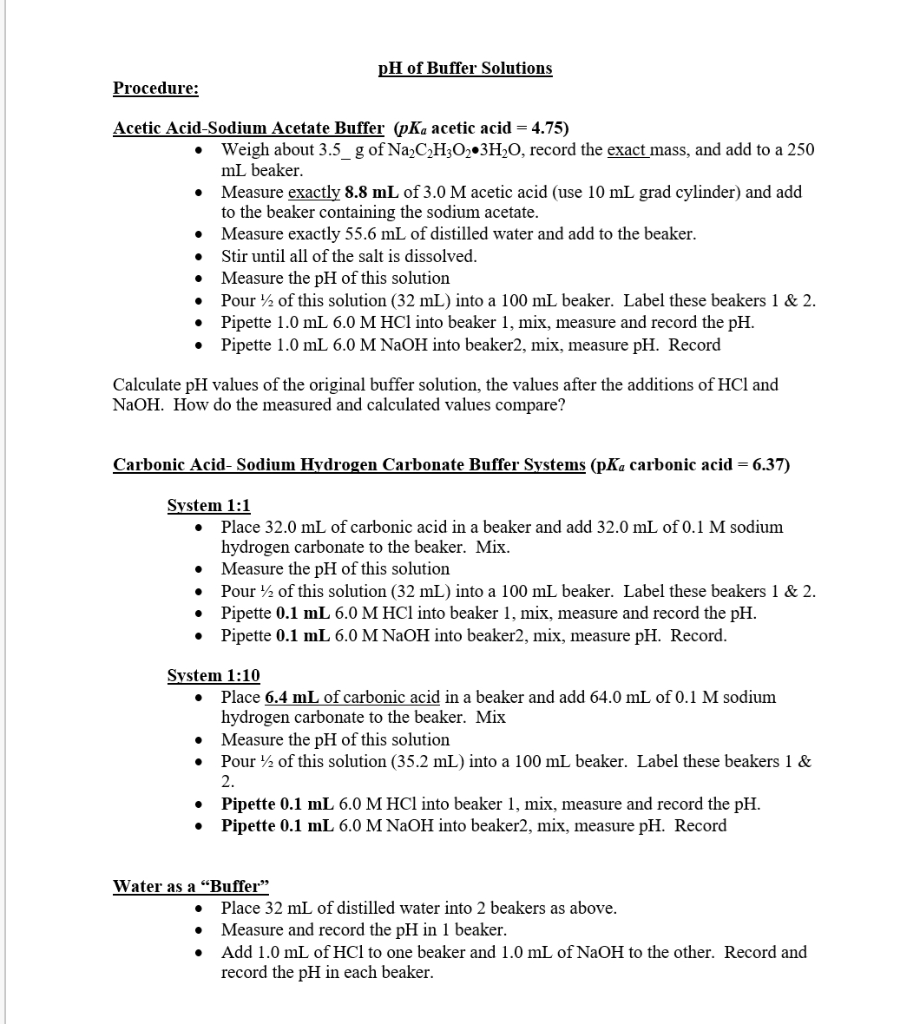Please help, I'm so confused!!!! This is due wednesday night!!! i'm gonna fail :(((( pH of Buffer Solutions Procedure: Acetic Acid-Sodium Acetate Buffer (pKa acetic acid = 4.75) Weigh about 3.5 g of Na2C2H302 3H2O, record the exact mass, and add to a 250 ml beaker. Measure exactly 8.8 mL of 3.0 M acetic acid (use 10 mL grad cylinder) and add to the beaker containing the sodium acetate. • Measure exactly 55.6 mL of distilled water and add to...